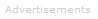# Definition of Electrical Resistance

What is Resistance?

Electrical resistance is opposition to the flow of current in an electric circuit: resistance converts electrical energy to thermal energy, and in this regard is similar to mechanical friction. Resistances are said to dissipate electrical energy as heat.### The Source of Resistance

Metals can be regarded as a lattice of positively charged metal ions surrounded by a 'sea' of mobile electrons that are not bound to any particular metal nucleus. These electrons are described as occupying the metal's conduction band.

When a potential difference - in other words a voltage - is applied to a metal in a circuit, it causes a net movement of electrons in the metal's conduction band.

The movement of electrons is hindered by vibration of the atoms in the metal lattice, which causes part of the electrical energy of the electric current to be lost - this is resistance. Since lattice vibrations increase as the temperature rises, the resistance of metals also increases as the temperature rises.

### Conductors and Insulators

Electrical conductors, such as metals, have low resistances. An ideal conductor would have zero resistance.

Electrical insulators have very high resistances. An ideal insulator would have infinite resistance: it would dissipate no energy, because no current could flow through it.

### Zero Resistance

At very low temperatures the resistance of some metals and materials fall to zero: current flows with no dissipation of electrical energy as heat. This phenomenon is called superconductivity.

### Ohm's Law

For many materials, electrical resistance, R, is defined by Ohm's law:

R = V / I

where V is voltage in volts and I is current in amps. The unit of resistance is the ohm, symbol Ω

For example, if a voltage of 9.0 V is applied to device and the current is measured to be 2.0 A, then the value of the resistance is 4.5 Ω.

Materials that obey Ohm's law are described as ohmic resistors. In general, metals are ohmic resistors and metalloid semi-conductors are not.

### Making use of Resistance

In the real world, electrical resistance can be utilized in consumer devices such as kettles, electric toasters, and immersion heaters to convert electrical energy to heat.

The electrical power output of a resistor is given by multiplying the (current through it) x (the voltage across it).

P = I V

where P is power, in watts, I is current in amps, and where V is voltage in volts.

### Resistance vs Impedance

The resistance of an ideal resistor is independent of the electricity's frequency. If the ratio of voltage to current changes with frequency, then the opposition to current is described as impedance rather than resistance.Search the Dictionary for More Terms